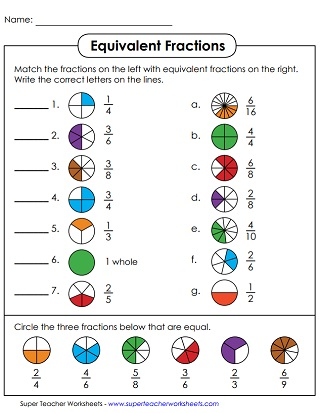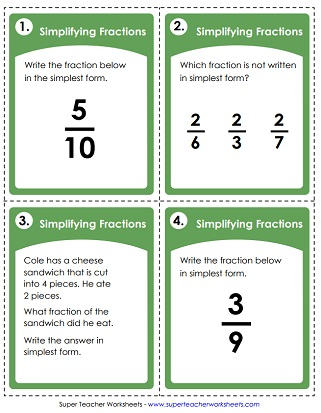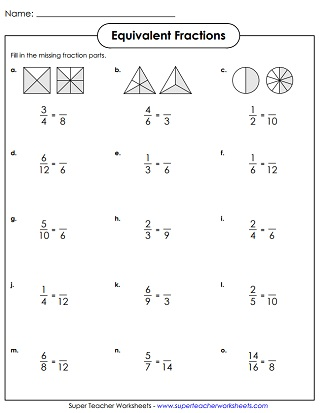# Equivalent Fractions & Simplifying Fractions

The worksheets below cover equivalent fractions and simplifying fractions. These printables are aligned with the following Common Core Standards: 3.NF.3, 3.NF.3a, 3.NF.3b, and 4.NF.1.## Equivalent Fractions

Use the illustrations/models to find equivalent fractions. All fractions on this sheet have denominators of 2, 3, 4, 6, and 8.
Find pairs of fractions that are equivalent in this memory match game. Cards have denominators between 2 and 15.
Fill in the missing fraction parts to make equivalent fractions. Denominators range from 2 through 16.
Part 1: Examine the models to figure out each pair of equivalent fractions. Part 2: Shade the correct portion of each polygon to show an equivalent fraction. Includes only denominators 2, 3, 4, 6, and 8.
Find equivalent fractions. This worksheet includes many graphical questions that help students to visualize the concept. Also, determine which fractions are in simplest form.
Cut the stars into 30 pieces and place them on a table. Match equivalent fractions to complete the star puzzles. (Note: Click the "alt" button below if you'd prefer a full-color version of this PDF.)
Match the fractions on the left with the equivalent fractions on the right. At the bottom, circle three fractions that are equal. This worksheet includes colorful circle illustrations.
Determine the correct fraction amounts to balance the scales.
Students can use the printable task cards to find equivalent fractions. Denominators range from 0 to 20.
This bookmark shows equivalent fractions, in order, from least to greatest.

## Simplifying Fractions

This worksheet has 5 parts. First, reduce fractions into simplest form. Then circle the fractions that are in simplest form. After that, answer two word problems. Then tell whether each equation is true or false.
This is a set of 30 task cards on simplifying fractions. They work great for learning centers, small group instruction, classroom scavenger hunts, peer tutoring sessions, and class games.
Simplify each fraction in this image. Then color the corresponding section according to the answer. Students will reveal a ladybug image as the completed math mystery picture.
Each of these word problems has a fraction answer that needs to be reduced, and written in simplest form.
Color half of each set. Then write a fraction to tell how many items were colored in.
Comparing & Ordering Fractions

Use the mathematical symbols <, >, and = to compare pairs of fractions. Also includes activities for ordering fractions from least to greatest and greatest to least.

More Fraction Worksheets

Link to sections of our site with worksheets on comparing fractions, fractions of sets, adding fractions, mixed numbers, reciprocal fractions, and more.

## Pictures of Our WorksheetsMy Account
Site Information